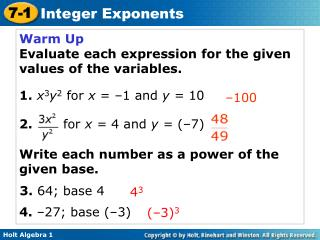Download PresentationWarm Up Evaluate each expression for the given values of the variables.

# Warm Up Evaluate each expression for the given values of the variables. - PowerPoint PPT PresentationDownload Presentation## Warm Up Evaluate each expression for the given values of the variables.

- - - - - - - - - - - - - - - - - - - - - - - - - - - E N D - - - - - - - - - - - - - - - - - - - - - - - - - - -
##### Presentation Transcript

1. Warm Up Evaluate each expression for the given values of the variables. 1.x3y2 for x = –1 and y = 10 2. for x = 4 and y = (–7) Write each number as a power of the given base. –100 3. 64; base 4 43 4. –27; base (–3) (–3)3

2. Learning Targets Students will be able to: Simplify & Evaluate expressions containing zero and integer exponents.

3.  5  5  5  5 You have seen positive exponents. Recall that to simplify 32, use 3 as a factor 2 times: 32 = 3  3 = 9. But what does it mean for an exponent to be negative or 0? You can use a table and look for a pattern to figure it out. 55 54 53 52 51 50 5–1 5–2 625 3125 125 25 5

4. Remember! Base x Exponent 4

5. One cup is 2–4 gallons. Simplify this expression.

6. Simplify. A. 4–3 B. 70 Any nonzero number raised to the zero power is 1. 7º = 1 C. (–5)–4 D. –5–4

7. Caution In (–3)–4, the base is negative because the negative sign is inside the parentheses. In –3–4 the base (3) is positive.

8. Simplify. a. 10–4 b. (–2)–4 c. (–2)–5 d. –2–5

9. Use the definition Evaluate the expression for the given value of the variables. x–2 for x = 4 Substitute 4 for x.

10. Evaluate the expression for the given values of the variables. –2a0b-4 for a = 5 and b = –3 Substitute 5 for a and –3 for b. Evaluate expressions with exponents. Write the power in the denominator as a product. Evaluate the powers in the product. Simplify.

11. Evaluate the expression for the given value of the variable. p–3 for p = 4 Substitute 4 for p. Evaluate exponent. Write the power in the denominator as a product. Evaluate the powers in the product.

12. for a = –2 and b = 6 Evaluate the expression for the given values of the variables. Substitute –2 for a and 6 for b. Evaluate expressions with exponents. Write the power in the denominator as a product. Evaluate the powers in the product. 2 Simplify.

13. B. Simplify. A. 7w–4

14. and Simplify. C.

15. Simplify. a. 2r0m–3 b. c. HWp.449/25-77odd, Day 2 P.450/78-97